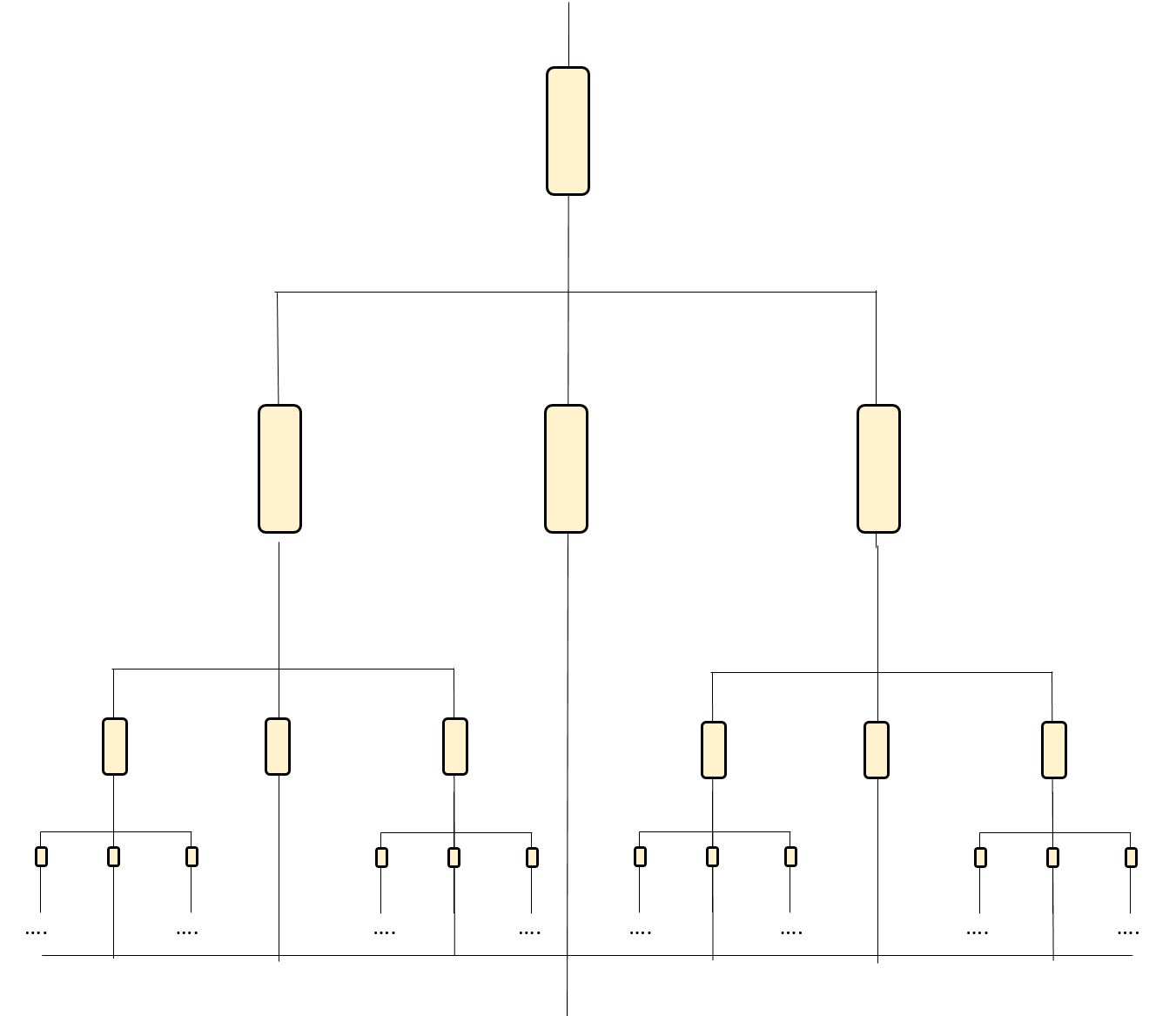# Cantor EquivalentIn the diagram above, each resistor has a value of 990 ohm. The recursive pattern of branching of the first and third branch continues infinitely.

Calculate the equivalent resistance of this circuit, rounded to the nearest integer.

×